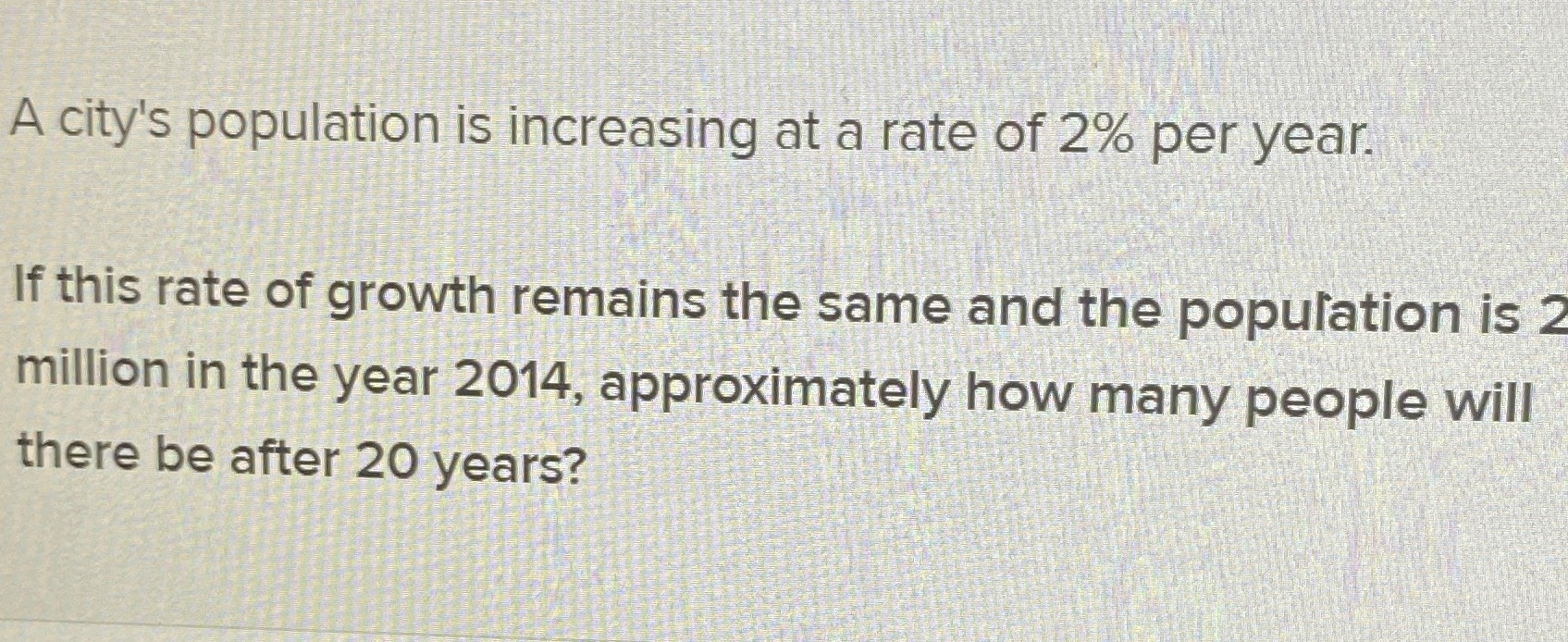### ¿Todavía tienes preguntas de matemáticas?

Pregunte a nuestros tutores expertos
Algebra
PreguntaA city's population is increasing at a rate of $$2 \%$$ per year. If this rate of growth remains the same and the population is $$2$$ million in the year $$2014$$ , approximately how many people will there be after $$20$$ years?